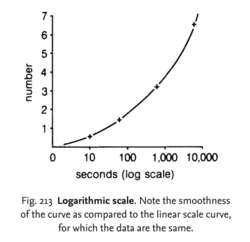# logarithmic scale

(redirected from Log scale)
Also found in: Dictionary, Thesaurus, Financial, Encyclopedia.Fig. 213 Logarithmic scale . Note the smoothness of the curve as compared to the linear scale curve, for which the data are the same.

## logarithmic scale

a scale in which the values of a variable are expressed as logarithms. Such transformations of data are often employed to simplify the drawing of lines on a graph, where the variable values are spread over a wide range. See Fig. 213 . A good example of the use of log scales is seen in Fig. 182 where the LOG PHASE of a microbial GROWTH CURVE is illustrated. Compare LINEAR SCALE.
Collins Dictionary of Biology, 3rd ed. © W. G. Hale, V. A. Saunders, J. P. Margham 2005
References in periodicals archive ?
The indirect estimate is obtained as the difference of the direct estimates of comparisons ACE versus BB and CCB versus BB in log scale. Furthermore, the direct and indirect estimates are pooled together to get the mixed estimate.
The slope on log scale algorithm performed poorly because the largest proportional rate-of-change for the number of cases tended to occur during periods of very low case numbers (perhaps reflecting chance fluctuations).
In comparison with the nonlinearity of the artist's laws of perspective drawing, the nonlinearity of the log scale is more abstract and less intuitive.
The ratios for, [[Beta].sub.0], [[Beta].sub.1], and the log scale parameter at the design stress are found.
Annual variation in addition to trend was modeled by multiplying the overall abundance by a random log-normal variable with mean (on the log scale) of 0 and variance set by a model parameter.
Because the distribution of values for urinary PdG and [E.sub.1]C were strongly skewed toward the upper end, we transformed daily urinary PdG and [E.sub.1]C to the log scale for analysis.
Looking at the figure "Mapping Sizes" (opposite), we see three levels of the log scale from left to right.
All commonly used hardwood log scales are more than a century old.
Mapped on the log scale, it comes into perspective.
Overrun refers to the difference between the actual volume of lumber produced by a mill and the log scale volume.
LO is the amount of lumber actually recovered in excess of the amount predicted by the log scale, expressed as a percentage of the log scale.

Site: Follow: Share:
Open / Close